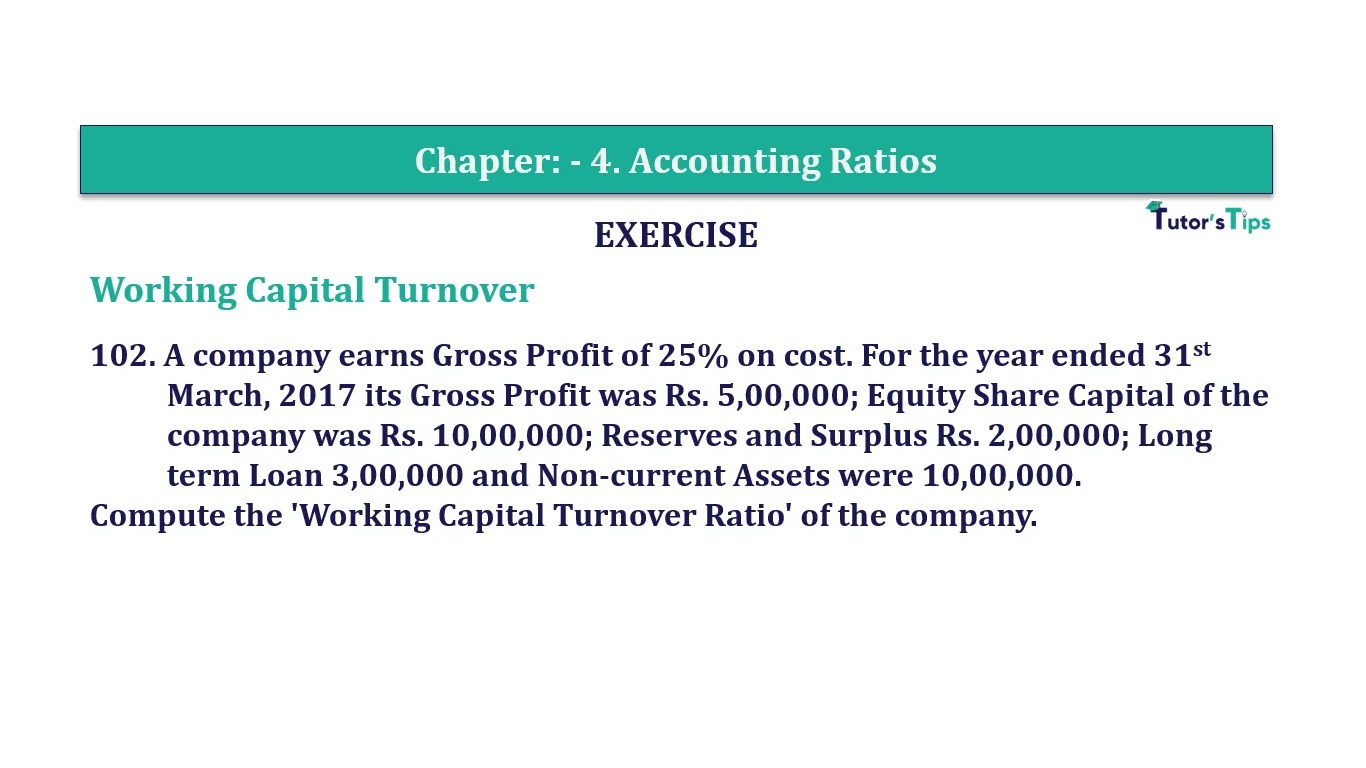# Question 102 Chapter 4 of +2-B – T.S. Grewal 12 ClassQuestion No. 102- Chapter No.4 - T.S. Grewal +2 Book Part B

Question 102 Chapter 4 of +2-B

Working Capital Turnover

102. A company earns Gross Profit of 25% on cost. For the year ended 31st
March, 2017 its Gross Profit was Rs. 5,00,000; Equity Share Capital of the
company was Rs. 10,00,000; Reserves and Surplus Rs. 2,00,000; Long
term Loan 3,00,000 and Non-current Assets were 10,00,000.
Compute the ‘Working Capital Turnover Ratio’ of the company.

### The solution of Question 102 Chapter 4 of +2-B: –

 Gross Profit = 25% on Cost Cost of Goods sold = 100 Gross Profit = 25 Revenue from Operations = 125
 Revenue from Operations = Rs.5,00,000 X 125 25 = Rs.25,00,000

 Capital Employed = Shareholder’s Funds + Non- Current Liabilities = Rs. 10,00,000 + Rs. 2,00,000 + Rs. 3,00,000 = Rs. 15,00,000 Working Capital = Capital Employed – Non- current Assets = Rs. 15,00,000 – Rs. 10,00,000 = Rs. 5,00,000

 Working Capital Turnover Ratio = Net Sales Working Capital
 = Rs. 25,00,000 Rs. 5,00,000 = 5 Times

Balance Sheet: Meaning, Format & Examples

Comment if you have any question.

Also, Check out the solved question of previous Chapters: –